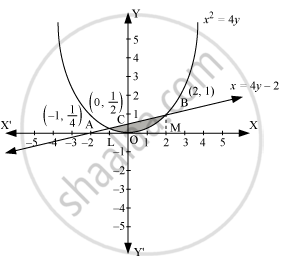# Using integration, find the area bounded by the curve x^2 = 4y and the line x = 4y − 2 - Mathematics

Using integration, find the area bounded by the curve x2 = 4y and the line x = 4y − 2.

#### Solution

The area bounded by the curve, x2 = 4y, and line, x = 4y − 2, is represented by the shaded area OBAO.Let A and B be the points of intersection of the line and parabola.

Coordinates of point A are (-1, 1/4).

Coordinates of point B are (2, 1).

We draw AL and BM perpendicular to x-axis.

It can be observed that,

Area OBAO = Area OBCO + Area OACO … (1)

Then, Area OBCO = Area OMBC − Area OMBO

=int_0^2(x+2)/4dx-int_0^2x^2/4dx

=1/4[x^2/2+2x]_0^2-1/4[x^3/3]_0^2

=-1/4[(-1)^2/2+2(-1)]-[1/4((-1)^3/3)]

=7/24

Therefore, required area = (5/6+7/24)=9/8 units

Concept: Area of the Region Bounded by a Curve and a Line
Is there an error in this question or solution?